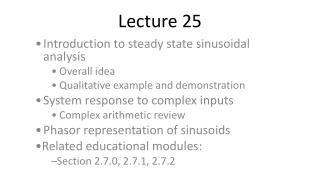DownloadDownload PresentationLecture 25

# Lecture 25

Download Presentation## Lecture 25

- - - - - - - - - - - - - - - - - - - - - - - - - - - E N D - - - - - - - - - - - - - - - - - - - - - - - - - - -
##### Presentation Transcript

1. Lecture 25 Introduction to steady state sinusoidal analysis Overall idea Qualitative example and demonstration System response to complex inputs Complex arithmetic review Phasor representation of sinusoids Related educational modules: Section 2.7.0, 2.7.1, 2.7.2

2. Steady state sinusoidal response – overview • We have examined the natural response and step response of electrical circuits • We now consider the forced response of a circuit to sinusoidal inputs • We will only consider the steady state response to the sinusoidal input • Apply a sinusoidal input and let t   • The steady state sinusoidal response • Corresponds to the particular solution

3. Why is this important? • Sinusoidal signals are very common • Power signals commonly sinusoidal (AC signals) • Carrier signals in communications often sinusoidal • The mathematics is considerably simpler • Differential equations become algebraic • System behavior often specified in terms of the system’s steady-state sinusoidal response • Example: Audio system specifications • It’s “natural” – our senses often work this way

4. System response to sinusoidal input • Apply a sinusoidal input, beginning at t = 0 • u(t) = Acos(t + ), t>0

5. Sinusoidal response of linear systems • The steady state response of a linear system, to a sinusoidal input, will be a sinusoid of the same frequency (particular solution of same form as input) • The amplitude and phase can change • These changes are, in general, a function of the frequency

6. - • Demo system response • Sinusoidal input to tower • Indicate response: transient, steady-state, frequency dependence

7. RL circuit steady state sinusoidal response • Apply a sinusoidal input to RL circuit: u(t) = Acos(t + ) • Governing equation (t):

8. - • Note on previous slide that di/dt is NOT zero for steady-state sinusoidal response!

9. Determining steady state sinusoidal responses • Obtaining solutions in terms of sines and cosines is tedious! • Try a “trick” involving complex exponentials: Acos(t + ) = Re{Aej(t+)} = Re{Aejejt} • Look at the response of the system to a complex exponential input, Aejejt • Results in a complex exponential response, Bejejt • The actual input is the real part of the complex input • The actual output is the real part of the complex output

10. - • Note: Complex exponentials previously discussed in lecture 21. • We’ll do a little review, but it may be worthwhile for you to review lecture 21, if you are insecure about complex exponentials – we’ll be using them a LOT now • Point out that complex input not physically realizable!

11. RL circuit response – revisited • Apply a complex exponential input: u(t) = Aej ejt • Governing equation (t) • Assume form of solution:

12. - • Annotate last bullet of previous slide, to show di/dt and where terms go in governing differential equation

13. RL circuit response to complex input • Substitute assumed solution into governing equation: • We can cancel ejt : • Since [Lej+R] is simply a complex number:

14. - • Note in previous slide: • In equation 1, we no longer have a differential equation – it’s algebraic! • In equation 2, the governing equation is no longer even a function of time! (The coefficients are, however, functions of frequency) • The drawback: complex numbers are now involved. (Point out in equation 2) • We will do a little complex arithmetic review in the next few slides.

15. Complex numbers – review • Rectangular coordinates: • Polar coordinates: • Relationships:

16. Review of complex arithmetic • Given two complex numbers: • Addition: • Subtraction:

17. Review of complex arithmetic – continued • Same two complex numbers: • Multiplication: • Division:

18. Review of complex arithmetic • Same two complex numbers, but in polar form: • Multiplication: • Division:

19. - • Annotate first “division” equation to note that 1/exp(phi) = exp(-phi)

20. Complex arithmetic – summary • Addition, subtraction generally easiest in rectangular coordinates • Add or subtract real and imaginary parts individually • Multiplication, division generally easiest in polar coordinates • Multiplication: multiply magnitudes, add phases • Division: divide magnitudes, subtract phases

21. Phasors • Recall: • Complex exponentials can be used to represent sinusoids • Complex exponentials can be written as a complex number multiplying a time-varying complex exponential • The complex number Aejprovides the magnitude and phase of the original sinusoidal signal

22. Phasors – definition • The complex number (in polar form) providing the magnitude and phase of a sinusoidal signal is called a phasor.

23. Example • Use phasors to determine the current i(t) in the circuit below if Vs(t) = Vmcos(100t).

24. - • In previous slide, show: • Change of input to complex exponential • Derivation of governing differential equation.

25. Example – continued • The governing differential equation is: • Since the input has the form , where is a phasor representing the input magnitude and phase, the output must be of the form , where is a phasor representing the output magnitude and phase.

26. - • In previous slide, emphasize concepts. Do substitution and write algebraic equation.

27. Example – still continued…

28. - • In previous slide, do complex arithmetic to solve for current phasor; convert back to time domain.

29. Example – Time domain signals • Input: • Response: • The response lags the input by 45